Study S4 Mathematics Vectors - Geniebook# Vectors

In the following article, we will be learning about Vectors as per the Secondary 4 Mathematics syllabus. We will cover the following sub–topics:

• Introduction to Vectors
• Representation of Vectors
• Vectors on a Cartesian Plane
• Magnitude of Vector
• Equal Vectors

## Introduction To Vectors

What is the difference between distance and displacement?

Let’s look at the below examples to understand these concepts

1. Anna walks 50 m along the path.
2. Anna walks 50 m along a path, travelling North.

Sentence 1 is an example of distance. Sentence 2 is an example of displacement. As we compare both the sentences, the units for both the sentences are the same, that is, 50 m.

In both the sentences, the magnitude is mentioned. The distance is given too, that is 50 m. However, in sentence 2, there is additional information given, that is, the direction in which Anna walks.

Therefore, the difference between distance and displacement is direction.

Hence, we realise that there are 2 types of quantities:

## Scalar And Vector

A $$vector$$ is a quantity with both $$magnitude$$ and $$direction$$, while a $$scalar$$ is a quantity $$with \;only \;magnitude$$.

Examples of Scalar Quantity are distance, speed, etc.

Examples of Vector Quantity are displacement, velocity, etc.

To Summarise:

• If an object has just magnitude, it is called a Scalar Quantity.
• If an object has magnitude and direction, it is called a Vector Quantity.

## Representation Of Vectors

A vector is represented by a $$directed \;line \;segment$$ where the length represents the $$magnitude$$ and the arrow represents the $$magnitude$$.The length from A to B is magnitude. The arrow represents the direction, which is upwards.The vector is denoted by $$\overrightarrow{AB}$$ or $$a̰\;$$ where A is the initial/starting point and B is the terminal/ending point.

## Vectors On A Cartesian Plane

Vectors can also be represented as a column vector,

$$\begin{pmatrix} x \\ y \end{pmatrix}$$

where $$x$$ represents the number of units along the horizontal axis

and $$y$$ represents the number of units along the vertical axis.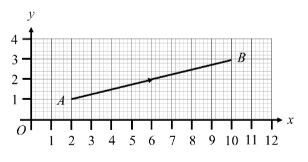Let’s say we have our vector $$\overrightarrow{AB}$$ on a cartesian plane.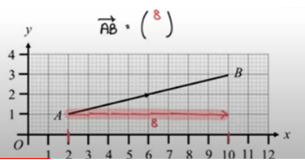For a column vector, the units along the horizontal axis (x axis) must be known.

In the cartesian plane above, the vector $$\overrightarrow{AB}$$ stretches from 2 units to 10 units on the x axis. The total units on the horizontal units are 8 units.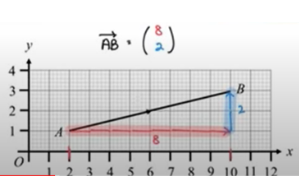On the vertical axis (y axis), we join the end point of the horizontal units to point B of the $$\overrightarrow{AB}$$ vector, in an up–down position. So, on the vertical axis, there are a total 2 units.

Therefore, we can say that $$\overrightarrow{AB} = \begin{pmatrix} 8 \\ 2 \end{pmatrix}$$

Question 1:

Write down the column vectors represented by $$\overrightarrow{AB}$$ , $$\overrightarrow{CD}$$ and $$\overrightarrow{EF}$$Solution: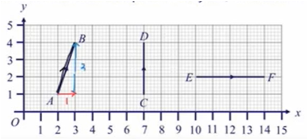$$\overrightarrow{AB} \; \begin{pmatrix} 1 \\ 3 \end{pmatrix}$$

From point A to point B, there is movement of 1 unit to the right on the x axis. There is a movement of 3 units on the y axis.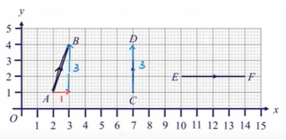$$\overrightarrow{CD} \; \begin{pmatrix} 0 \\ 3 \end{pmatrix}$$

When there is no movement either on right or left, then the unit is 0. There is no movement on the x axis, hence, it is 0 units. From point C to D there is upwards movement of 3 units on the y axis.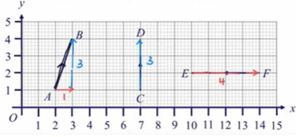$$\overrightarrow{EF} \; \begin{pmatrix} 4 \\ 0 \end{pmatrix}$$

From point  E to  F, there is a rightward movement of 4 units. There is no upwards or downwards movement on y axis, so the unit is 0.

Question 2:

Draw the label clearly the vectors $$\overrightarrow{AB} \; \begin{pmatrix} 3 \\ -4 \end{pmatrix}$$ and $$\overrightarrow{CD} \; \begin{pmatrix} -1 \\ 5 \end{pmatrix}$$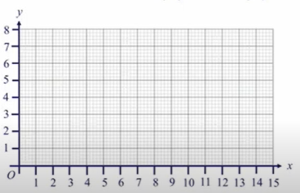Solution: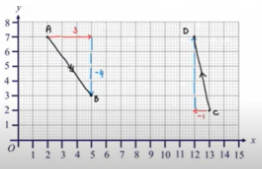In the cartesian plane, at point A, the  positive 3 units are moved along the x axis  and negative –4 is moved along the y axis. So, point B will end up where –4 has been marked completely. So, on joining point A and B will give the $$\overrightarrow{AB}$$.

The point C is –1 unit is leftwards at the x axis.. Then there are  5 units along the y-axis. The point D is where the 5 units end. On joining point C and D, it gives the $$\overrightarrow{CD}$$

## Magnitude Of A Vector

The magnitude of a vector is denoted by $$|\overrightarrow{AB}|$$  or $$|a̰|$$ and can be calculated using Pythagoras Theorem.

\begin{aligned} |\overrightarrow{AB}| = a = \sqrt {x^2 + y^2} \;,\; where\; \overrightarrow{AB} = a̰ = \begin{pmatrix} x \\ y \end{pmatrix} \end{aligned}.

Question 3:

Find the magnitude of vectors $$\overrightarrow{AB}$$ , $$\overrightarrow{CD}$$ and $$\overrightarrow{EF}$$.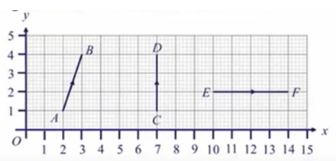Solution: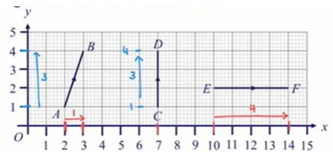We will first find the column vector of $$\overrightarrow{AB}$$.

$$\overrightarrow{AB} = \begin{pmatrix} 1 \\ 3 \end{pmatrix}$$

\begin{aligned} |\overrightarrow{AB}| &= \sqrt {(1)^2 + (3)^2} \\ \\ &= \sqrt {10} \\ \\ &= 3.1622 \\ \\ &\approx 3.16 \end{aligned}

We will first the column vector of $$\overrightarrow{CD}$$

$$\overrightarrow{CD} = \begin{pmatrix} 0 \\ 3 \end{pmatrix}$$

\begin{aligned} |\overrightarrow{CD}| &= \sqrt {(0)^2 + (3)^2} \\ \\ &= \sqrt {9} \\ \\ &= 3 \\ \\ \end{aligned}

We will first find the column vector of $$\overrightarrow{EF}$$

$$\overrightarrow{EF} = \begin{pmatrix} 4 \\ 0 \end{pmatrix}$$

\begin{aligned} |\overrightarrow{CD}| &= \sqrt {(4)^2 + (0)^2} \\ \\ &= \sqrt {16} \\ \\ &= 4 \\ \\ \end{aligned}

Question 4:

Given that $$c̰ = \begin{pmatrix} m \\ -3 \end{pmatrix}$$ , find the possible value(s) of m if $$|\;c̰\;|=5$$ units.

Solution:

Application of the vector formula first:

$$|\;c̰\;|=5$$

\begin{aligned} |\;c̰\;| &= \sqrt {(m)^2 + (-3)^2} \\ \\ \implies \;\;\;5 &= \sqrt {(m)^2 + 9} \\ \\ \implies \;\;5^2 &= {(m)^2 + 9} \\ \\ \implies \;\;25 &= {(m)^2 + 9} \\ \\ \implies \;m^2 &= {25 - 9} \\ \\ \implies \;m^2 &= {16} \\ \\ \implies \;\;m &= 4 \;\;\; or \; -4 \\ \\ \end{aligned}

## Conclusion

The article introduces what is a vector and discusses the representation of vectors and magnitude of vectors. Further, it also displays the vectors on the cartesian plane.

Continue Learning
Sets: Venn Diagrams, Intersections & Union Probability of Combined Events
Statistical Data Analysis Matrices
VectorsPrimaryPrimary 1Primary 2Primary 3Primary 4Primary 5Primary 6SecondarySecondary 1Secondary 2Secondary 3Secondary 4PhysicsChemistryMathsE-Maths
Sets: Venn Diagrams, Intersections & Union
Probability of Combined Events
Statistical Data Analysis
Matrices
VectorsA-Maths